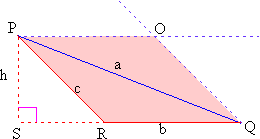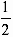Home MonkeyNotes Printable Notes Digital Library Study Guides Message Boards Study Smart Parents Tips College Planning Test Prep Fun Zone Help / FAQ How to Cite New Title Request

 4.5 Triangle Just as the area of parallelogram was derived from the area of rectangle, the area of a triangle can be derived from the area of a parallelogram. Consider the triangle PQR (figure 4.4). If a line is drawn through P parallel to RQ and another line is drawn through Q parallel to PR they will intersect at O. POQR is a parallelogram with PQ as its diagonal.Figure 4.4 Recall that the diagonal of a parallelogram divides the parallelogram into two congruent triangles. \ Area of parallelogram POQR = 2 ´ Area D PQR or Area D PQR =Area of parallelogram POQR. Area of parallelogram POQR = bh where h is the altitude on the base with length h. \ Area D PQR =bh Area of a triangle is half the product of one base and the corresponding altitude. The perimeter of the triangle is simply the sum of all its sides. P = ( a + b + c ) in figure 4.4. Index 4.1 Perimeter 4.2 Square 4.3 Rectangle 4.4 Parallelogram 4.5 Triangle 4.6 Trapezoids 4.7 Circles Chapter 5
 Search: All Products Books Popular Music Classical Music Video DVD Toys & Games Electronics Software Tools & Hardware Outdoor Living Kitchen & Housewares Camera & Photo Cell Phones Keywords: